## CPU Core Vs Threads: Utilization Differences...Part 2

Posted on 13-May-2011 by Craig Shallahamer, craig@orapub.com

This is so typical... What I think will be a casual research project ends up being so much more. I hope you find this blog entry very enlightening and useful in your Oracle Database performance work.

### The Purpose

In part one of this series I mentioned in a few rare occurrences I have seen a utilization mismatch. That is, there is a difference between the utilization calculated using the busy time and CPU core count from Oracle Database view v\$osstat compared to the utilization gathered from the OS vmstat. While I have not seen this very many times, it does occur and so I wanted to do a more formal investigation to:

Check the difference is statistically different. (It could be the result of randomness.)

Is this something I need to be concerned about in my performance analysis work, and

Understand how a mismatch could be possible.

It's questions like these I wanted to gain a firmer grasp...based on real production Oracle data, not through a thought experiment, talking to the OS administrator or vendor, or reading from a blog or book. So here we go...

This is a fairly long entry; full of experimental and analysis details. If you want to skip all the details, just scroll down to the final section, "Summary" and read only that.

### Utilization References

Throughout this blog entry when I write "Oracle utilization," I am referring to the average CPU utilization calculated over a time interval as follows:

U = ( busy_time delta from v\$osstat ) / ( interval time X CPU cores from v\$osstat )

The CPU cores from v\$osstat can be determined by taking the largest non-thread CPU count-type statistic value. If cores are not mentioned, then use a CPU statistic. I know this is strange, but I've never seen this approach fail (yet).

When I refer to "OS utilization," I am referring to the average CPU utilization gathered over a time interval using the OS command vmstat.

If these formulas seem strange, please refer to Part 1 of this series.

### The Experiment

To accomplish my goals I needed utilization related data from real Oracle production systems. So I created a data collection script and asked a bunch of DBAs to run it on their production systems.

The Script.

The script is nothing special. It's a simple shell script with some awk and greps, a little math and there is even a while loop. The inputs are the number of samples to collect and the snapshot interval time. I gathered and calculated the CPU utilization two different ways. First, based on the busy_time and CPU cores from the data source v\$osstat. Second, I also gathered the CPU utilization from the OS using vmstat. The results for each sample were written to an output file and lots of details were written to a log file as well. I wrote the script to work on Linux. It is very well documented and explains what is likely to need modification to work on any Unix-like OS. Click to view the Linux version.

The Participants and Data.

I sent emails to a couple folks who commonly will collect data for me from their production systems. I also sent about fifty other emails to some students from my recent classes (pictures). From my thinking, I had a great response. As you personally know DBAs are extremely busy plus I'm asking them to run a script in one of their production systems...it's a lot to ask.

But I did get some data and it is goooood data. As I mentioned, my analysis is based on seven sample sets from HPUX, Linux, and AIX environments. There were no outliers and no data was removed. All the data was placed directly in the Mathematica Notepad and you can view the data by simply looking at the resulting PDFs (see below). Each sample set consisted of 60 samples, so I was well above my chosen minimum of 30. Each sample interval was 15 minutes; long enough to remove any significant collection timing error but not too long to miss significant utilization swings.

By the way, I do a lot of these types of experiments. If you want to participate, just send me an email (start by sending to orapub@comcast.net). If you desire, I will keep you anonymous, which I have done with everyone in this experiment.

### The Analysis

The Process

To analyze the data, I used Mathematica. As you can see by looking at the output, it's very clean and allows me to document the analysis in detail within the Mathematica "Notebook." All the data has been placed into the single Mathematica notebook. The data is at the bottom of the notebook and it is documented with specifics such as the OS and number of cores and threads. I also provide the participate's reference code (so I can go back and reference all the emails, raw data files, etc.).

The analysis notebook contains five main sections; Background and Purpose, Data Loading, Basic Statistics, Utilization Difference Analysis, and the Experimental Data. You can view each of the analysis results, on-line in a PDF file, by clicking on the links below.

1. AG1. AIX, 16 cores, each with 2 threads (32 total threads).

2. AG2. Linux, 4 cores, each with 4 threads (16 total threads).

3. GO1. HPUX, 3 cores, each with 2 threads (6 total threads).

4. LZ1. HPUX, 32 cores, each with 2 threads (64 total threads).

5. LZ2. HPUX, 32 cores, each with 2 threads (64 total threads).

6. NN1. Solaris, 4 cores, each with 2 threads (8 total threads).

7. RB1. Linux, 8 cores, not sure if threads are being used.

To download the "analysis pack" (in a single zip file) which contains all the above analysis PDFs, Mathematica notebook (think of the notebook as the source file), and the data collection script, click here.

### The Raw Results

Figure 1 below is a summary of the results.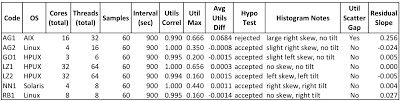Here is a short explanation of each figure column.

Code is the code to identify the sample set and to keep the data provider anonymous.

OS is the operating system.

Cores (total) is the total number of CPU cores.

Samples is the number of experimental sample collected and used in the analysis.

Interval (sec) is the sample duration for every collected sample.

Utils Correl is the correlation coefficient relating the relationship strength between the two utilizations; v\$osstat core based and OS vmstat based. A high correlation indicates when one of the utilizations changes so does the other. A correlation coefficient in the 0.90+ is very high, so as you can see all of our samples showed a staggeringly high correlation.

Util Max is the maximum utilization of any sample value.

Avg Util Diff is the Oracle utilization (v\$osstat core based) minus the OS utilization (vmstat). Throughout this analysis, the difference is always calculated this way.

Hypo Test is the result of hypothesis test (alpha=0.05), testing if the utilization differences are enough indicate they came from different sources, such as different utilization calculations or even a different database server. All but one of the samples easily passed the hypothesis test. There is more information about the hypothesis test below and also in the Mathematica notebooks.

Histogram Notes are simply short observations regarding the histogram of the utilization differences. A nice looking "bell curve" indicates the error hovers around the mean and there is not a significant and harmful trend.

Util Scatter Gap shows the utilization values for all of our samples; both Oracle based and OS based. Therefore, there are 120 datapoints on the graph. This graph allows us to quickly and visually tell if there are significant differences in the utilization calculations and also when they occurred. For example, we can visually tell if these gaps increase as the utilization increases. That is a harmful trend we will want to know about.

Residual Slope is the slope of the linear trend line based on the utilization error (i.e., difference) as the y-axis and the Oracle based utilization as the x-axis. This is another method to spot a harmful trend. A harmful trend example is when the utilization increases so does the difference between the two utilization sources. If the slope is zero (completely flat) this means regardless of the utilization the difference is always the same. The line could be flat but above the zero y-axis, which indicates while the utilization difference is the same regardless of the utilization, the Oracle utilization is always greater than the OS based utilization. Residual graphs are a powerful way to look for harmful trends.

### Summary of Results

I could write on and on about the individual samples, but it's not necessary (thankfully). All but one of the samples (AG1) are very similar. All the non AG1 samples visually and mathematically indicate while there is a little difference in the utilization calculations, it is very small, consistent over the gathered utilization range, and the difference in the utilizations is normally distributed.

The only concern I have is the none of the sample sets were gathered from a system with the CPU utilization greater than 65%. I would like to see some sample sets with a CPU constrained system. It is possible if the utilization differences are highly skewed and the residual slope is not flat (discussed below), the utilization difference (i.e., the gap) could become increasingly larger. This stresses the importance of understanding how utilization is calculated and what this truly means for our Oracle production systems. While I will write below about the practical value of the analysis, the How Can This Be? will be posted in my next entry...it's just too much information for a single blog entry.

### Detailed Results (Selected)

I'm going to review in detail two sample sets. The two are very different and will make a nice contrast; LZ1 and AG1.

LZ1 Sample Set

I chose to focus on the LZ1 sample set because the CPU subsystem is relatively active (max 66%), it's a massive database server (64 cores), and is HPUX based. You can view the PDF analysis file by clicking here. But I have extracted some of the graphics and show them below.

First, there is a strikingly strong and positive correlation (1.000) between the two utilizations. This means when one of the utilizations increases so will the other...nearly always. So while there is a slight difference between the two utilization calculations, they are very highly correlated.

This slight difference in utilizations is indeed small...infinitesimal is a better word. The average difference is only 0.00028 of a percentage point...basically no difference.

A tiny utilization calculation difference may not seem or feel significant, but statistically is might be too much to indicate the data sets are the result of two different calculations. This is another of saying we want to test if our two sample sets are statistically different. If they are different, then we know any difference is not caused by randomness. The difference could be caused any number of things, but not randomness.

But itʼs a little more complicated then is usual... We canʼt expect all the Oracle utilization data values to be normally distributed. Think about it; there could easily be two clusters of values, say around 20% and 50% busy. This would result in a non-nomal distribution of utilization values. The same is true for the vmstat utilization data values. Because of this non-normality, we canʼt perform a simple t-test.

All is not lost. According to the central limit theorem (reference one and two) if the differences in the sample pairs are normally distributed then we can statistically say the samples came from the population. Relating this to utilization, if I gather 100 vmstat utilization samples (the population), divide the 100 samples into two 50 sample sets, create a new third sample set based on the differences in the samples ( S51-S1, S52-S2,..., S100-S50 ), the new sample set will be normally distributed. So cool---this occurs regardless of the distribution of the initial two sample sets. ...I see another blog entry coming...

So this is what I did: I created a new sample set based on the differences between each collection method (one value for Oracle based utilization and the other value from vmstat). If the new "differences" sample set is normally distributed, than any differences in the samples (e.g., S51-S1) do exist, the difference can attributed to randomness. On the flip-side, if the new sample set is not normally distributed, we know the utilization differences are not caused by randomness...but by something else.

Back to the LZ1 analysis: I performed a significant test (alpha=0.05) to see if the "differences" sample set is so different that randomness could not account for the difference and therefore the difference was caused by something else...like perhaps different utilization calculations. As expected, the two sample sets are similar enough (Anderson-Darling test, p=0.950) to indicate any difference is caused by randomness.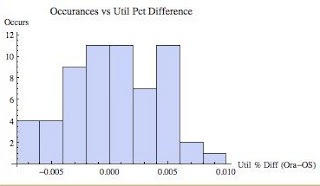Figure 2 above is the histogram based on the "differences" sample set. The histogram doesn't look perfectly normal; it's kind of flat near (negative kurtosis) the top and the left tail is "fat." However, it's not that bad (I realize that's not real scientific.) What I like to see if the error tappers off at the tails, so that it is centered around the average...so we're close.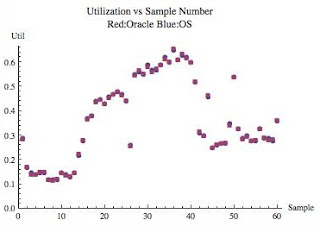Figure 3 above is a scatter plot of the two utilizations, not the "differences." All 60 samples are represented and for each sample there is an Oracle utilization point (red square) and an OS utilization point (blue circle). In the figure it is difficult to distinguish the two points because they are almost the same! This means the utilization calculations are pretty much the same. But even more important; the gap is very consistent the same regardless of the sample or the utilization.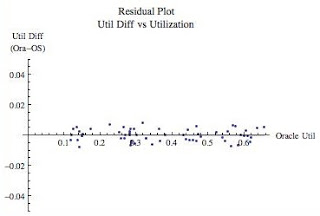Figure 4 above is known as a residual plot and is a phenomenal way to spot trends in the error, that is, the differences in the utilization calculations. It is common for mathematical models to work wonderfully at lower utilizations but get increasingly poor as the utilization increases. (We tackle how to remove the error in my Oracle Forecasting and Predictive Analysis course.) The residual graph is one way to visually help us detect if this is occurring. For our purposes, we want to check visually again (like we did with the scatter plot above) if the utilization difference is greater based on the utilization. If the trend line of the residuals is flat (and it is with a slope of 0.0002331) then the utilization difference does not change based on the utilization...for the samples we selected. You probably can't even see the trend line because it is virtually on the x-axis. I could have made the y-axis range smaller and effectively stretch the graph top to bottom, but I want to fair comparison with the AG1 data set I'll detail below.

To summarize sample set LZ1, both utilization calculations always resulted in nearly the same value, the difference did not change as the utilization increased, and the hypothesis test showed the differences in the utilization can be explained by randomness. Based on this information, I am comfortable using either utilization calculation until signifiant CPU queuing begins, which will occur at around 85%. Then I would gather additional data and re-analyze.

AG1 Sample Set

The Oracle based utilization peaked at 68%, so we have an active CPU subsystem. The AG1 sample set is unique compared to the others because from multiple perspectives there is a clear difference in the utilization calculations. Just by looking at the average difference, Oracle v\$osstat core based utilization calculation is 6.8% higher than vmstat reports. But it gets more interesting...

There is a strikingly strong and positive correlation between the two utilization sources. This means when one of the utilizations increases so will the other...nearly always. So while there is a near 7% difference in the utilization sources, they are still very highly correlated.

A 7% difference may seem or feel significant, but statistically is might not be a big deal and could have been caused by randomness. Just as with the LZ1 sample set, I performed a significance test (alpha=0.05) to see if the utilization difference in each sample pair are significantly different. As we suspected, statistically speaking there is significant difference (Anderson-Darling test, p=0.040). This means the difference can't be explained purely by randomness and something else is causing the difference...perhaps it's the difference in utilization calculations or something else.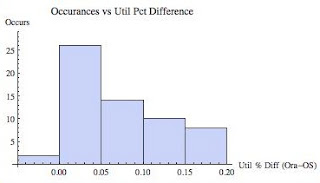Figure 5 is a histogram of the utilization differences from our sample. Notice on all but two occasions, the Oracle utilization was higher than the OS (vmstat) utilization. We can also visually see the utilization differences are not normally distributed but heavily skewed to the right (skew>0, skew is positive)...in fact it looks log normal.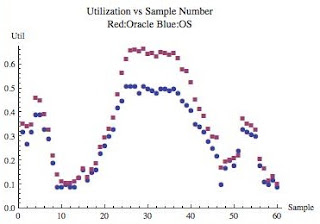Figure 6 above is very cool! It clearly shows three things; First, the utilization trends nicely from as low as 10% to over 65%. Second, we can clearly see the high utilization samples result in a larger difference between the utilization sources. And finally, the Oracle utilization (v\$osstat core based) is nearly always greater than the OS vmstat based utilization. One would assume if the utilization increased further (say 90%) the utilization gap would continue to increase...but how do we know this? Read on...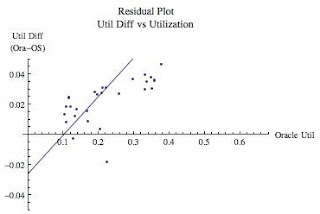Figure 7 above is the residual plot showing as the Oracle utilization increases, so does the difference between the utilization calculations. Compared to the other sample residual graphs, the slope looks shockingly steep! The trend line may not appear to match the data. It is because all the data is not shown to allow for a direct and fair comparison with the other sample sets (e.g., Figure 4 above).

Let's take a look at what this really means. The linear trend line slope is 0.2563. This means for every single percentage of Oracle utilization increase there is an additional 1/4 of a percentage point difference in the utilization calculations. Figure 7 shows when the Oracle utilization is around 35% (i.e., 0.35) there difference in utilizations is around 3%. If we extend the line to where the Oracle utilization is 60%, the difference in utilizations is expected to be around 13% (just plug the Oracle utilization number into the trend line formula, shown on the analysis PDF file). That is still not horrible, but not great either. The question now becomes, which utilization is the "real" utilization. This is the subject for my next blog entry.

To summarize sample set AZ1, all our tests indicate there is a significant utilization calculation difference between Oracle core-based and OS based CPU utilizations. When the utilization peaked, the Oracle based calculation was around 10% greater than vmstat showed and this difference is expected to increase as the utilization increases! So we had better decide which utilization source to use or we and everyone we work with could be confused. In the next blog entry I'll drill down into this topic.

### Summary

If you are concerned about which utilization method to use, don't worry...do a little analysis. You don't need to do a full-on analysis like I did. Just gather some data (you can even use my data collection script) and create a scatter plot like I did in Figure 3 and Figure 6. If you see a difference of more than around 5% then you may want to determine why the difference exists and also which method is relevant for your work.

Here are a few take-aways:

Can we use v\$osstat as a source to reliably calculate OS CPU utilization? Absolutely.

Can the v\$osstat core based utilization differ significantly from vmstat? Absolutely.

How do I know if I need to be concerned? As I mentioned directly above, collect some data and do a quick analysis. If you'd like, I can help QA your conclusions.

I think an even more intriguing question is why can there be differences in the utilization calculations between Oracle and the OS. That's the topic of my next blog entry...